# jenkins-report-generic-chart-column

Generic reusable plugin that will show a chart in column based on properties file.

The plugin reads properties file in your archive, specified by glob, and use one value, defined by key, to draw a chart for both project and view. The plugin was originally designed to show results of benchmarks, but can be misused for anything key-number what desire chart. Eg total and failed tests summaries, watching over size of package and so on. The graph is scaled, so you will never miss smallest change.

## Properties file

To make plugin work, you need a properties file with results form your job, archived. The properties file is eg.:

``````lastSuccessfulBuild/artifact/jbb-report/result/specjbb2015-C-20180717-00001/report-00001/specjbb2015-C-20180717-00001.raw
``````
``````# garbage
jbb2015.result.metric.max-jOPS = 22523
jbb2015.result.metric.critical-jOPS = 8902
jbb2015.result.SLA-10000-jOPS = 4774
jbb2015.result.SLA-25000-jOPS = 7442
jbb2015.result.SLA-50000-jOPS = 9643
jbb2015.result.SLA-75000-jOPS = 11833
jbb2015.result.SLA-100000-jOPS = 13791
other garbage
``````

The parser is quite forgiving, and will skip garabge. Supports both : and = delimiters.

## Project summary

Hugest graphs are shown in project summary. You can have as much graphs as you wish, and have detailed tool-tip: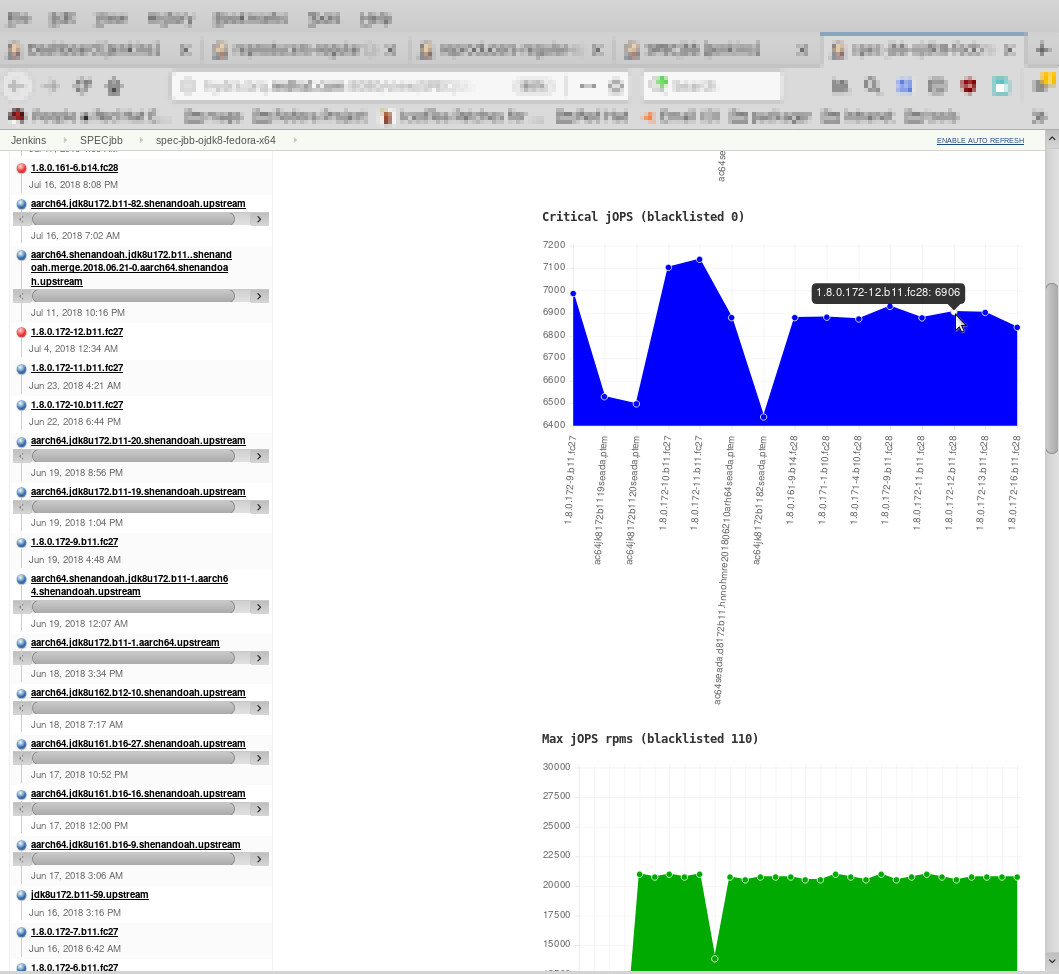Comparing individual jobs was never more simple:)

## View summary

You can include the graphs to the view: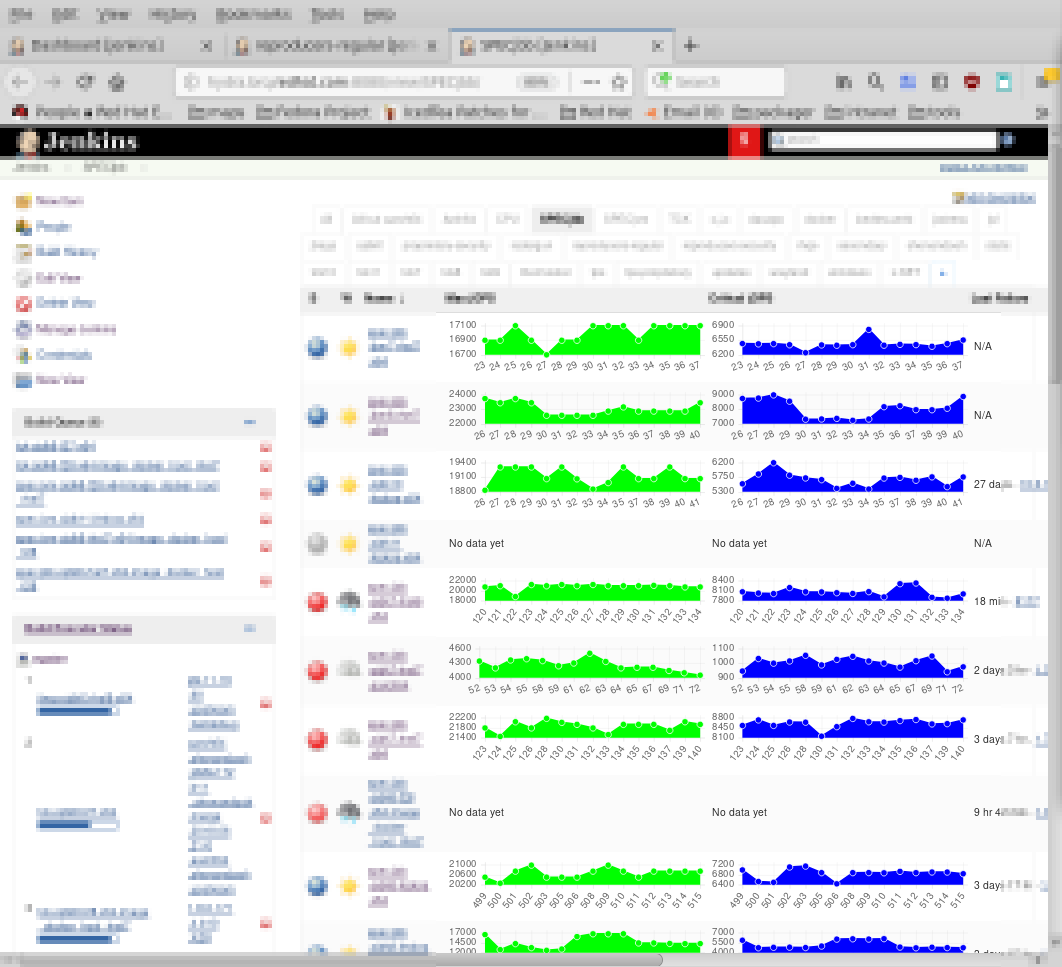Comparing individual projects was never more simple:)

You can of course mix it with other properties or other plugins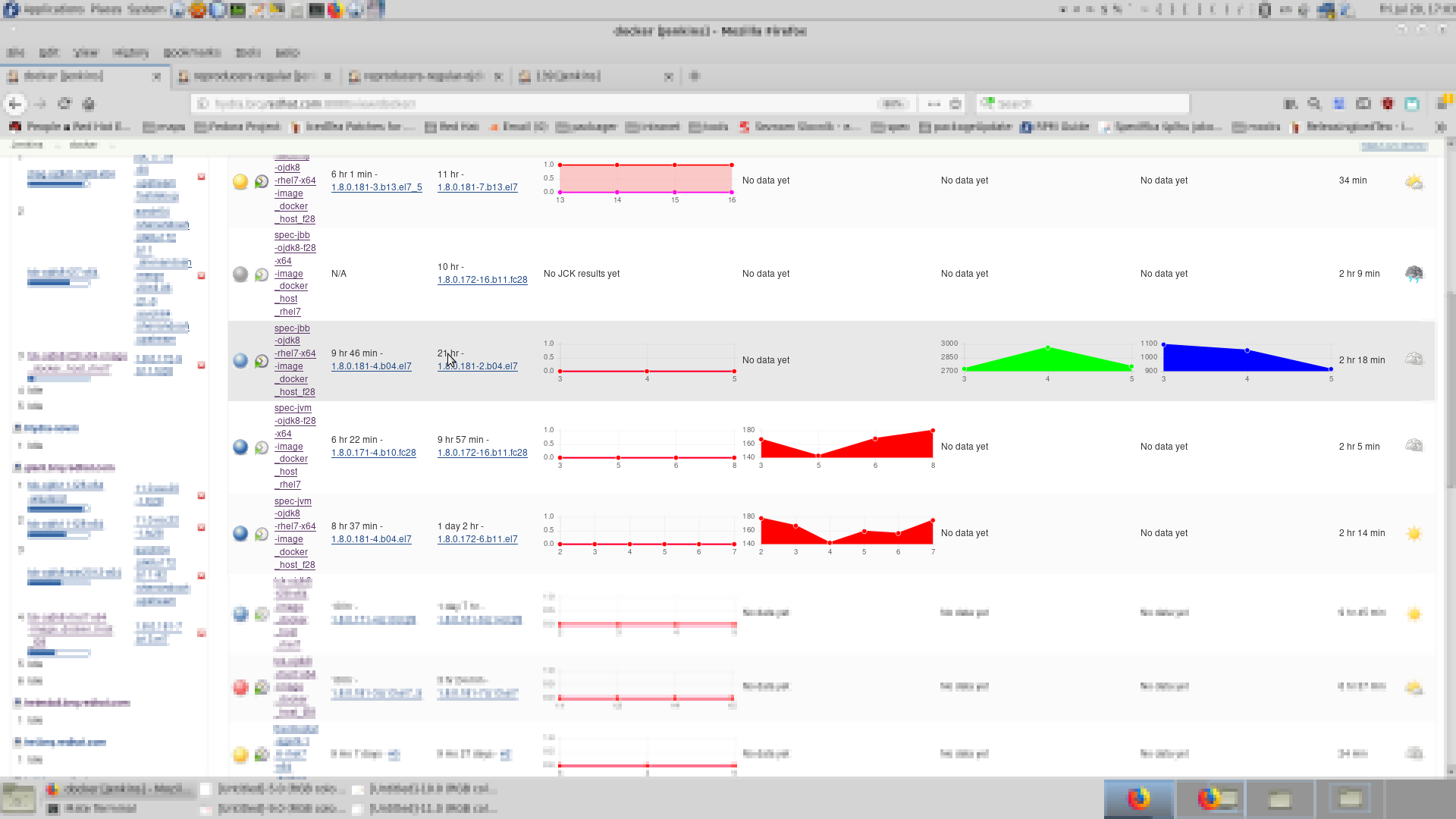The results in view are sort-able - they are sort by last valid result shown in chart.

Comparing individual projects was never ever more simple:)

## Changing build result

Each chart (there can be several by project) can have its own condition, on which result it can turn the build to unstable, if the condition is met. The mathematic part is handled by https://github.com/gbenroscience/ParserNG/, the logic part is internal. Exemplar expression:

``````avg(..L1)*1.1 <  L0 | L1*1.3 <  L0
``````

The expression can be read as: If value of key in last build is bigger then average value of all builds before multiplied by 1.1 , or the last build is bigger then previous build multiplied by 1.3, turn the build to unstable

The built which just ended is L0. Previous build is L1 and so on... You can use ranges - eg L5..L1 will return values of given key for build N-5,N-4-N-3,N-2,N-1 where N is current build - L0. Ranges can go withot limit - eg L3.. will exapnd as L3,L2,L1,L0. So obviously mmost used is ..L1 which returns you values of all except latests (L0) build. Count of points is MN.

The L indexes, can be calcualted. To do so, use `L{expression}`. Eg L{MN/2} upon 1,2,3, will expand as L{3/2} -> L{1,5} -> L1 -> 2. The brackets can be cumulative (eg L{{1+1}}) and can contain Other L or MN. eg L{L0}. The only reason why this was created was to beable wrote ..L{MN/2} and L{MN/2}..

### testing the expression

Next to the cmdline/library of ParserNG where you can try yours expressions, you can do similarly with jenkins-report-generic-chart-column.jar; but it is not exactly straightforward to compose classpath. eg:

``````VALUES_PNG="1 2 3" java  -cp jenkins-report-generic-chart-column.jar:parser-ng-0.1.8.jar  io/jenkins/plugins/genericchart/math/ExpandingExpression "sum(..L0) < avg(..L0)"
``````

or

``````VALUES_PNG="1 2 3" java  -cp parser-ng-0.1.8.jar:jenkins-report-generic-chart-column.jar  parser.ExpandingExpression  "avg(..L{MN/2}) < avg(L{MN/2}..)"
``````

or

``````VALUES_PNG="1 2 3" java  -cp jenkins-report-generic-chart-column.jar:parser-ng-0.1.8.jar  io/jenkins/plugins/genericchart/math/ExpandingExpression "sum(..L0) < avg(..L0)"
``````

Currently all necessary changes were moved to ParserNG, including the `VALUES_PNG` variable. ParserNG have powerfull CLI and since `0.1.9` this expanding parser is here, so you can run it simply as java -jar:

``````VALUES_PNG='235000 232500 233000 236000 210000' java parser-ng-0.1.9.jar -e " echo(L{MN}..L0) "
``````

or via its interactive CLI

``````\$ VALUES_PNG='235000 232500 233000 236000 210000' java -jar parser-ng-0.1.9.jar -e -i
``````
Output
``````Welcome To ParserNG Command Line
Math Question 1:
______________________________________________________
echo(L0..L{MN})
______________________________________________________
210000 236000 233000 232500 235000
Math Question 2:
______________________________________________________
echo(L{MN}..L0)
______________________________________________________
235000 232500 233000 236000 210000
``````
``````VALUES_PNG='235000 232500 233000 236000 210000' java -jar parser-ng-0.1.9.jar -e -i -v
``````
Output
``````Welcome To ParserNG Command Line

Math Question 1:
______________________________________________________
L1<L2
L1<L2
Expression : L1<L2
Upon       : 235000,232500,233000,236000,210000
As         : Ln...L1,L0
MN         = 5
Expanded as: 236000<233000
236000<233000
brackets: 236000<233000
evaluating logical: 236000<233000
evaluating comparison: 236000<233000
evaluating math: 236000
is: 236000
evaluating math: 233000
is: 233000
... 236000 < 233000
is: false
is: false
false
is: false
______________________________________________________
``````

### Most common expressions

#### Divergence from exact pivot

If something should be some exact result, or must not be an exact result is most easy usage

• `L0 == 5` if last result is 5, then the job will become unstable
• `L0 != 5` which is same as
• `![L0 == 5]` if last result is NOT 5, then the job will become unstable

#### Immediate regression:

• `threshold=5;-1*(L1/(L0/100)-100) < -threshold` which is same as
• `threshold=5; (L1/(L0/100)-100) > threshold` for classical benchmark, like score, where more is better. The threshold is how much % is maximal drop it can bear, and
• `threshold=5; (L1/(L0/100)-100) < -threshold` for eg size (where smaller is better) benchmark, or time-based where less is better . The threshold is how much % is maximal increase it can bear.
• For stable things 5% should be the biggest regression rate. For unstable once usually 10% is OK to cover usual oscillation
• Note, that those equation works fine for both big numbers and small numbers

#### Short term regression

Such last run against previous run can not catch constant degradation. To avoid that you may simply extends of Immediate regression, only `L0` will compared against all previous runs - L1 will become something lile `..L1` (all except last run)

You can then call `avg` or `avgN` functions above it or `geom` or `geomN` if you have to diverse data with huge thresholds. See parserNG help for descriptions of functions (you can type `help` also to the Jenkins settings for this equation)

• `threshold=5;-1*(avg(..L1)/(L0/100)-100) < -threshold` which is same as
• `threshold=5; (avg(..L1)/(L0/100)-100) > threshold` for classical benchmark, like score, where more is better. The threshold is how much % is maximal drop it can bear, and
• `threshold=5; (avg(..L1)/(L0/100)-100) < -threshold` for eg.: size (where smaller is better) benchmark, or time-based where less is better . The threshold is how much % is maximal increase it can bear.

#### Longer term regression

• In basic comparison, you can compare any Lx with any Ly. Eg `(L2/(L0/100)-100) > threshold` or `(L{MN}/(L0/100)-100) > threshold` and so on.
• The underlying evaluation is lenient, and eg L4 in size in set of two numbers, will have simply value of last valid (second in this case) number.
• That's also why `L{MN}` works, although you should be explicitly writing `L{MN-1}`
• example: ` VALUES_PNG='3 2 1' java -jar parser-ng-0.1.9.jar -e "echo(L5,L6,L7)"` will give you `3 3 3`
• another,more generic solution, may achieved by simply extension of Immediate regression, only `L0` will be replaced by something like `L0..L{MN/2}` (newer half of the set) and L1 by `L{MN/2}..L{MN}` (older half of the set)
• You can then call `avg` or `avgN` functions above it or `geom` or `geomN` if you have to diverse data with huge thresholds. See parserNG help for descriptions of functions (you can type `help` also to the Jenkins settings for this equation)
• `threshold=5;-1*(avg(L{MN/2}..L{MN})/(avg(L0..L{MN/2})/100)-100) < -threshold` which is same as
• `threshold=5; (avg(L{MN/2}..L{MN})/(avg(L0..L{MN/2})/100)-100) > threshold` for classical benchmark, where more is better. The threshold is how much % is maximal drop it can bear
• `threshold=5; (avg(L{MN/2}..L{MN})/(avg(L0..L{MN/2})/100)-100) < -threshold` for eg time-based or size benchmark, where less is better. The threshold is how much % is maximal increase it can bear.

#### Gluing it all together

You usually have more expressions which are catching your regressions, to connect them, you can use logical operators:

``````java -jar parser-ng-0.1.9.jar -l   "help"
Comparing operators - allowed with spaces:!=, ==, >=, <=, <, >; not allowed with spaces:le, ge, lt, gt,
Logical operators - allowed with spaces:, |, &; not allowed with spaces:impl, xor, imp, eq, or, and
As Mathematical parts are using () as brackets, Logical parts must be grouped by []
``````

Eg:

• for classical benchmarks like score:
``````threshold=5;-1*(L1/(L0/100)-100) < -threshold || threshold=5;-1*(avg(L{MN/2}..L{MN})/(avg(L0..L{MN/2})/100)-100) < -threshold || threshold=5;-1*(avg(..L1)/(L0/100)-100) < -threshold
``````
• for inverted benchmarks like time or size
``````threshold=5;   (L1/(L0/100)-100) < -threshold || threshold=5;   (avg(L{MN/2}..L{MN})/(avg(L0..L{MN/2})/100)-100) < -threshold || threshold=5;   (avg(..L1)/(L0/100)-100) < -threshold
``````

Note, that if the variables (eg my threshold above) are filled as they come to end. If you set it in first logical half, it can be reused in second without declaring it again (as I did in above example). Unluckily, you usually have thresholds different. You can re-declare (as I did) the variable or have different one (eg thresholdA and thresholdB) by ParserNG rules.

'avgN' and 'geomN' are usually producing better results, as they are getting rid of random extreme spikes by sorting the input, and removing `N lowest` and `N highest` values. N is first parameter. `avgN(0,...)` is identical to simply `avg(...)`:

``````VALUES_PNG='5 5 1 8 5 5'  java -jar parser-ng-0.1.9.jar -e "avgN(0,..L0)"
4.833333333
``````

but

``````VALUES_PNG='5 5 1 8 5 5'  java -jar parser-ng-0.1.9.jar -e "avgN(1,..L0)"
5
``````

as 8 and 1 were removed from list. So:

• for classical benchmarks like score:
``````cut=2;threshold=5;-1*(L1/(L0/100)-100) < -threshold || threshold=5;-1*(avgN(cut,L{MN/2}..L{MN})/(avgN(cut,L0..L{MN/2})/100)-100) < -threshold || threshold=5;-1*(avgN(cut,..L1)/(L0/100)-100) < -threshold
``````
• for inverted benchmarks like time or size
``````cut=2;threshold=5;   (L1/(L0/100)-100) < -threshold || threshold=5;   (avgN(cut,L{MN/2}..L{MN})/(avgN(cut,L0..L{MN/2})/100)-100) < -threshold || threshold=5;   (avgN(cut,..L1)/(L0/100)-100) < -threshold
``````

Is what yoy usually end with

#### Named queries

As it maybe boring and error prone to keep repeating compelx equations, you can set the equation in the global settings and then just call it via its name - even with different parameters.

There are same named queries already embedded: https://github.com/jenkinsci/report-generic-chart-column-plugin/blob/master/src/main/resources/io/jenkins/plugins/genericchart/presetEquations

`````` # FIRST_LINE_IS_ALWAYS_NAME
# then soem documentation
Then, on multiple lines without hash (as parserng -t)
there is the expression  with /*1*/ /*2*/
upto /*9*/ as placeholders for your arguments

# empty line then ends up the expression.
# you ca then call FIRST_LINE_IS_ALWAYS_NAME arg1 arg2 ...
``````

Well see examples and dont forget you can set up yor own in settings.

## Denylist and Allowlist

you could noted, that the graphs are scaled. If you have run, which escapes the normality, the scale get corrupted, and you can easily miss regression. To fix this, you have denylist (and allowlist). This is list of regexes, which filters (first) out and (second) in the (un)desired builds. It works both with custom_built_name and `#build_number` (note the hash). Empty denylist/allowlist means it is not used at all.

## Project Settings

Project settings and view settings are separate - with both pros and cons!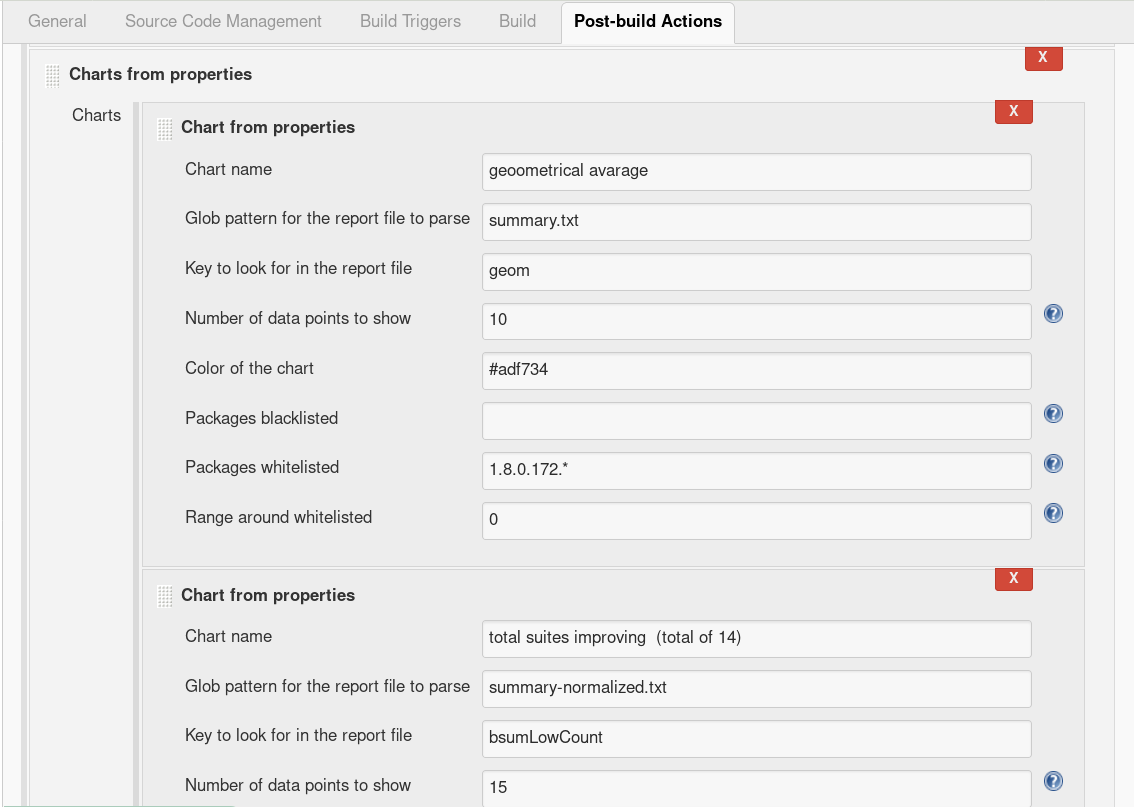Most important is Glob pattern for the report file to parse, which lets you specify not absolute (glob) path to your properties file and of course Key to look for in the report file which tells chart what value to render. Chart name and color are cosmetic, denylist and allowlist were already described. Number of data points to show is how many successful builds (counted from end) should be displayed. If you are in doubts, each suspicious field have help.

## View Settings

Project settings and view settings are separate - with both pros and cons!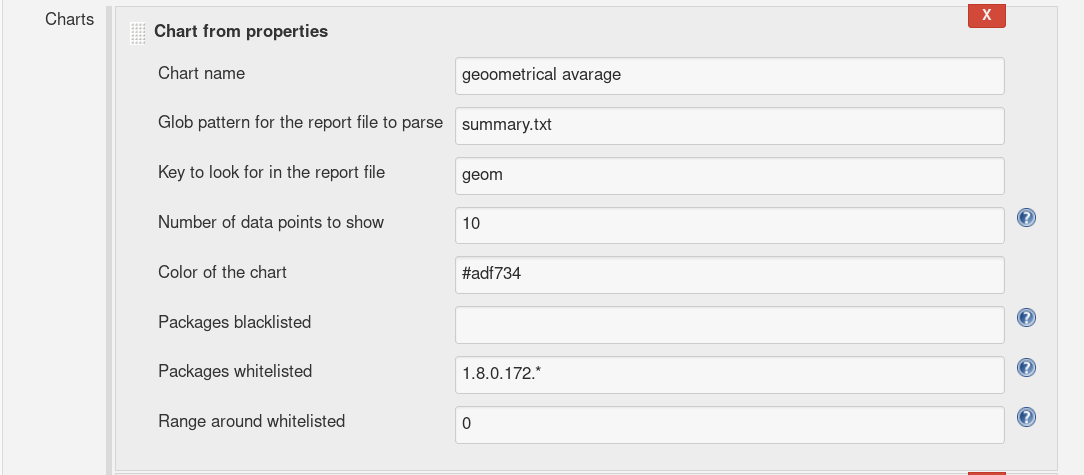You can see that the settings of view are same - thus duplicated with all its pros and cons...

## Limitations

The limitations flows from double settings and from fact that each chart can show only only one value. The non-shared denylist/allowlist is a negative which we are working on to improve. One line only is considered as - due to scaled graph - definitely positive.

## Range around allowlisted

Number of points before and after chosen point using allowlist. For example if you have allowlisted 3 and 4 and range is 2 graph will show points 1 2 3 4 5 6.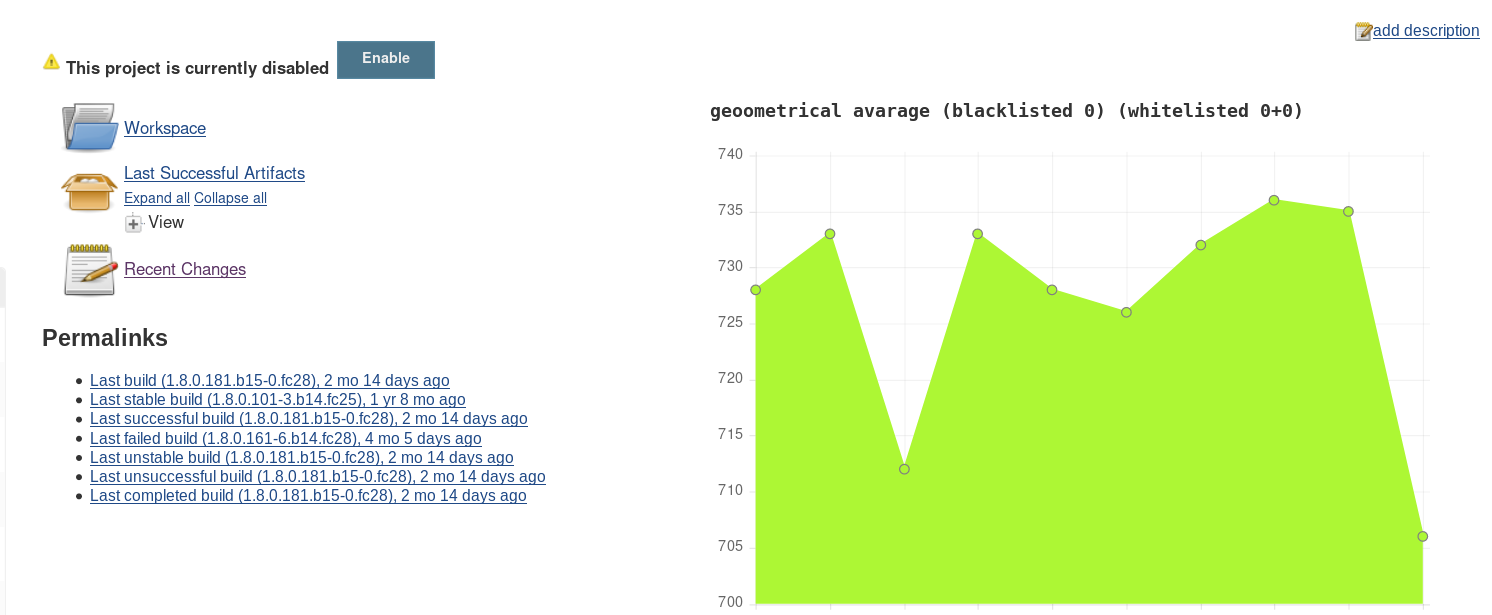Up is without allowlisted and range. Down is with allowlist (1.8.0.172.*) and range (3).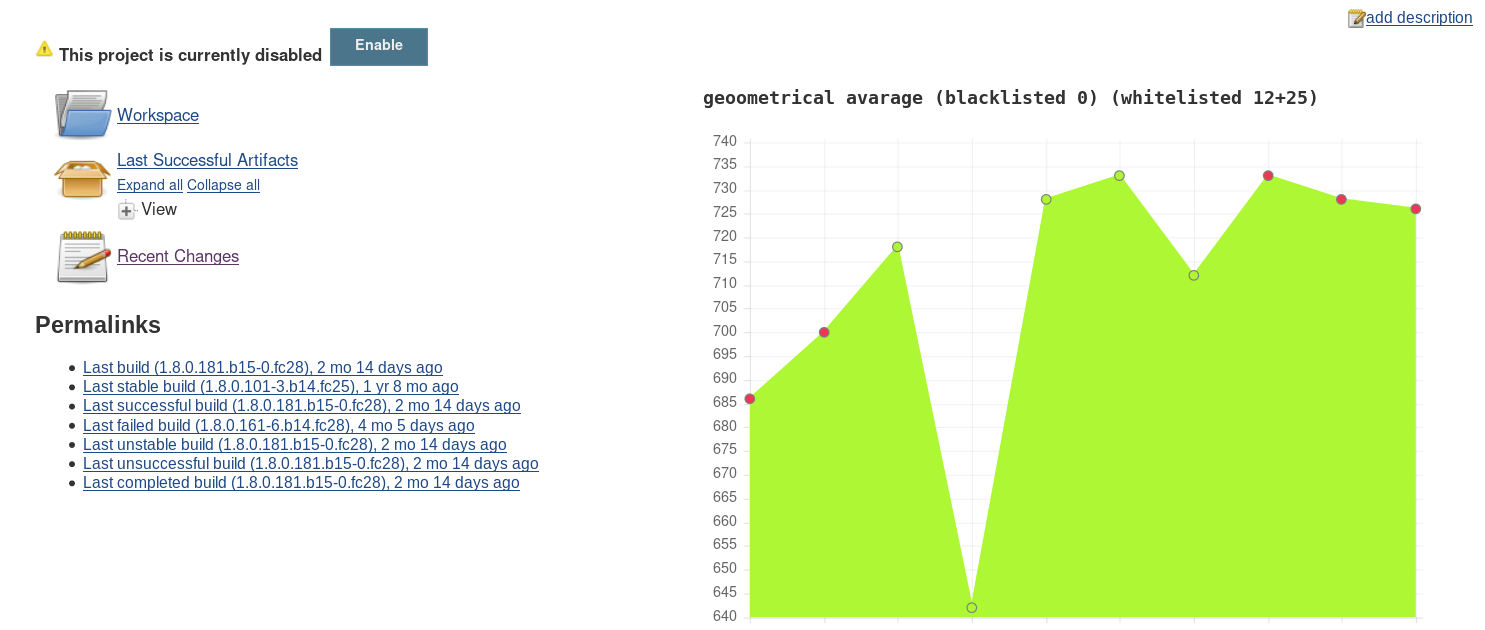Up is with allowlist (1.8.0.172.*) and range (3). Down is with allowlist (1.8.0.172.*) and without range.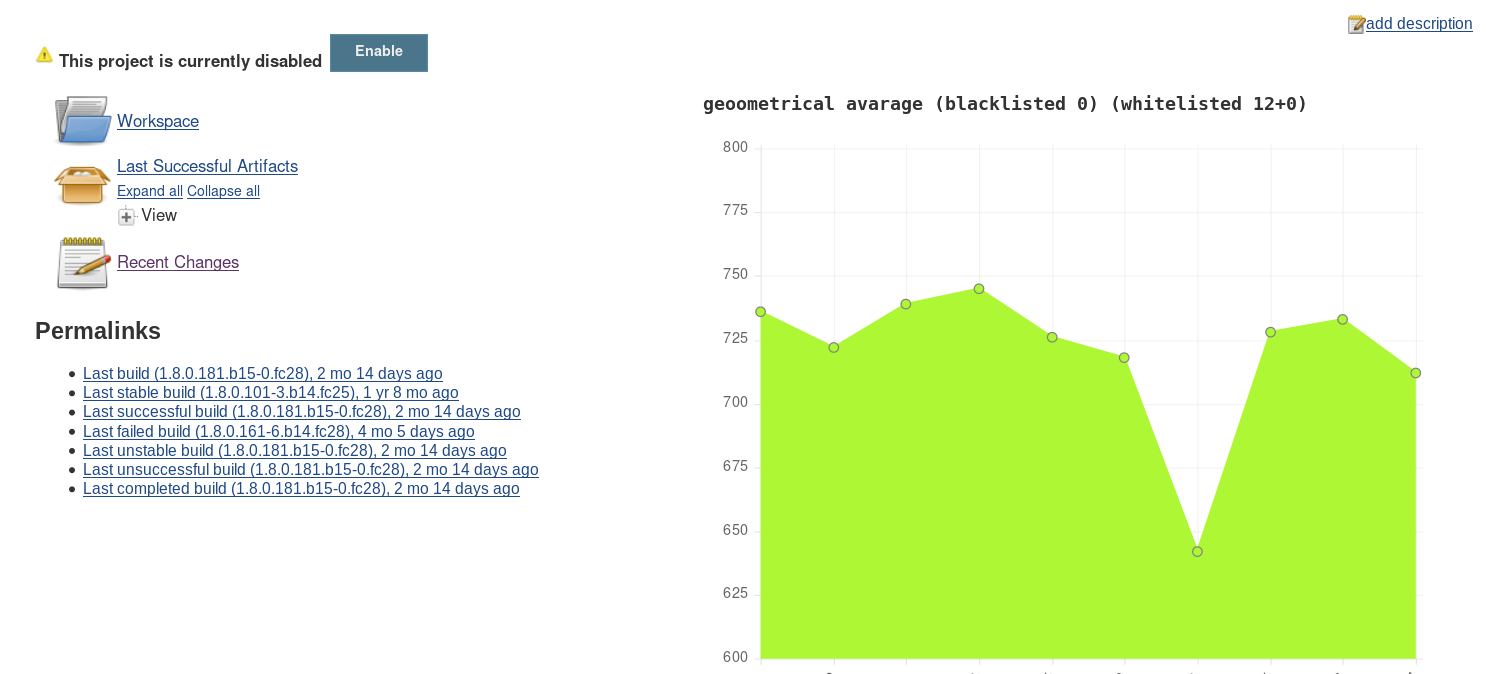## Future work

We wish to improve allowlist/denylist feature, so it can be used to generate views comparing selected runs across jobs with some kind of neighborhood

# Dependencies

This plugin depends on chartjs-api library plugin and on parser-ng math library 1.9 or up